Question

# A loop of wire has the shape shown in the drawing. The top part of the...

A loop of wire has the shape shown in the drawing. The top part of the wire is bent into a semicircle of radius r = 0.15 m. The normal to the plane of the loop is parallel to a constant magnetic field (ϕ = 0°) of magnitude 0.85 T. What is the change ΔΦ in the magnetic flux that passes through the loop when, starting with the position shown in the drawing, the semicircle is rotated through half a revolution?

given data is radius r=0.15m,magnitic field B=0.85T,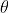1=00,2=1800(f0r half revolution)

change in magnetic flux ΔΦ =AB(cos1-cos2)

here A=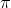r2/2=3.14*(0.15)2/2

ΔΦ=(3.14*(0.15)2/2)*0.85(cos00-cos1800)=0.06 weber

#### Earn Coins

Coins can be redeemed for fabulous gifts.

Similar Homework Help Questions
• ### A loop of wire has the shape shown in the drawing. The top part of the...

A loop of wire has the shape shown in the drawing. The top part of the wire is bent into a semicircle of radius r = 0.27 m. The normal to the plane of the loop is parallel to a constant magnetic field (ϕ = 0°) of magnitude 0.84 T. What is the change ΔΦ in the magnetic flux that passes through the loop when, starting with the position shown in the drawing, the semicircle is rotated through half a...

• ### 5. A loop of a wire has the shape shown in the drawing. The top part...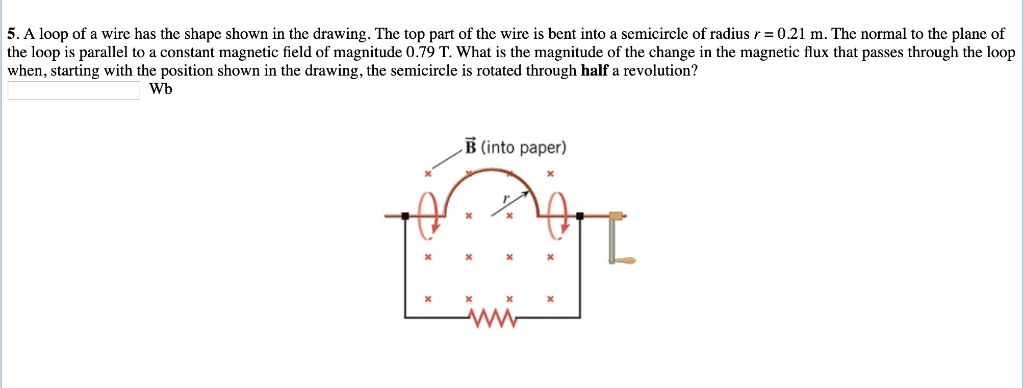5. A loop of a wire has the shape shown in the drawing. The top part of the wire is bent into a semicircle ofradius r = 0.21 m. The normal to the plane of the loop is parallel to a constant magnetic field of magnitude 0.79 T. What is the magnitude of the change in the magnetic flux that passes through the loop when, starting with the position shown in the drawing, the semicircle is rotated through half a...

• ### thank you in advance for your help! A magnetic field passes through a stationary wire loop,...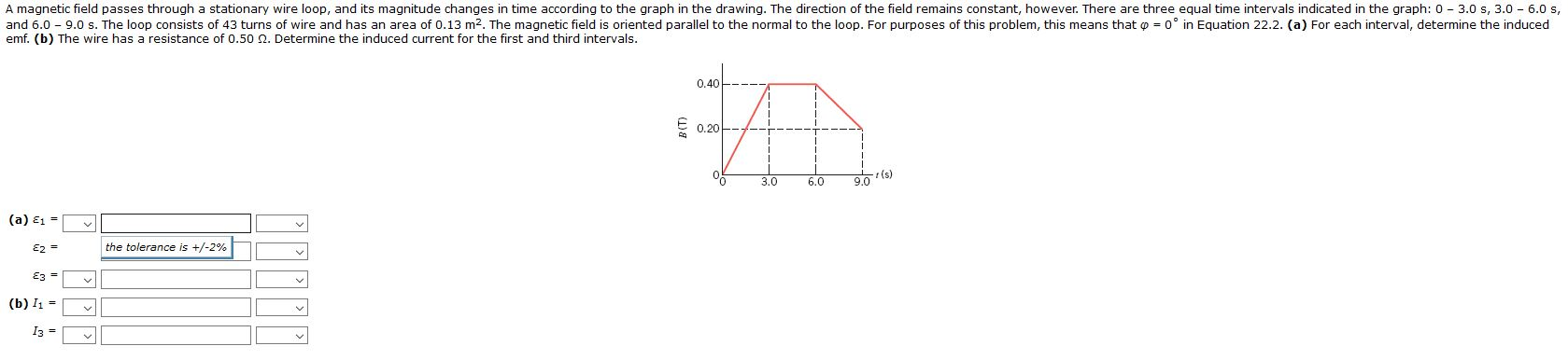thank you in advance for your help! A magnetic field passes through a stationary wire loop, and its magnitude changes in time according to the graph in the drawing. The direction of the field remains constant, however. There are three equal time intervals indicated in the graph: 0 - 3.0 s, 3.0 - 6.0 s, and 6.0 - 9.0 s. The loop consists of 43 turns of wire and has an area of 0.13 m2. The magnetic field is oriented...

• ### A 144-Ω light bulb is connected to a conducting wire that is wrapped into the shape...

A 144-Ω light bulb is connected to a conducting wire that is wrapped into the shape of a square with side length of 83.0 cm. This square loop is rotated within a uniform magnetic field of 454 mT. What is the change in magnetic flux through the loop when it rotates from a position where its area vector makes an angle of 30° with the field to a position where the area vector is parallel to the field? A 144-Ω...

• ### A rectangular loop of wire 24 cmcm by 72 cmcm is bent into an LL shape,...

A rectangular loop of wire 24 cmcm by 72 cmcm is bent into an LL shape, as shown in the figure. (Figure 1) The magnetic field in the vicinity of the loop has a magnitude of 3.8×10−2 TT and points in a direction 25 ∘∘ below the y axis. The magnetic field has no x component. Find the magnitude of the magnetic flux through the loop.

• ### A rectangular loop of wire 24 cm by 72 cm is bent into an L shape,...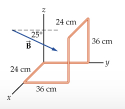A rectangular loop of wire 24 cm by 72 cm is bent into an L shape, as shown in the figure. (Figure 1) The magnetic field in the vicinity of the loop has a magnitude of 3.6×10−2 T and points in a direction 25 ∘ below the y axis. The magnetic field has no x component. 1. Find the magnitude of the magnetic flux through the loop. 24 cm 36 em 24 cm 36 cm

• ### A loop of wire with radius r=0.015 m is in a magnetic field with magnitude B as shown in the figure.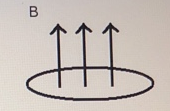A loop of wire with radius r=0.015 m is in a magnetic field with magnitude B as shown in the figure. B changes from B1 = 0.35 T to B2 = 4.5T in Δt=5.5s at a constant rate. The resistance of the wire is R=5Ω.Part (a) Express the magnetic flux going through a loop of radius r assuming a constant magnetic field B. Part (b) Express the magnetic flux change, 40, in terms of B1, B2, and r. Part (c) Calculate the...

• ### A uniform magnetic field passes through a horizontal circular wire loop at an angle 60° from...

A uniform magnetic field passes through a horizontal circular wire loop at an angle 60° from the normal to plane of the loop. The magnitude of the magnetic field is 3.0 T, and the radius of the wire loop is 10 cm. Find the magnetic flux through the loop.

• ### A uniform magnetic field passes through a horizontal circular wire loop at an angle 16.2° from...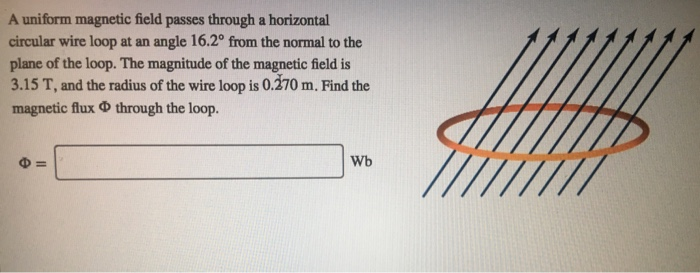A uniform magnetic field passes through a horizontal circular wire loop at an angle 16.2° from the normal to the plane of the loop. The magnitude of the magnetic field is 3.15 T, and the radius of the wire loop is 0.270 m. Find the magnetic flux ? through the loop.

• ### A uniform magnetic field passes through a horizontal circular wire loop at an angle 19.5° from...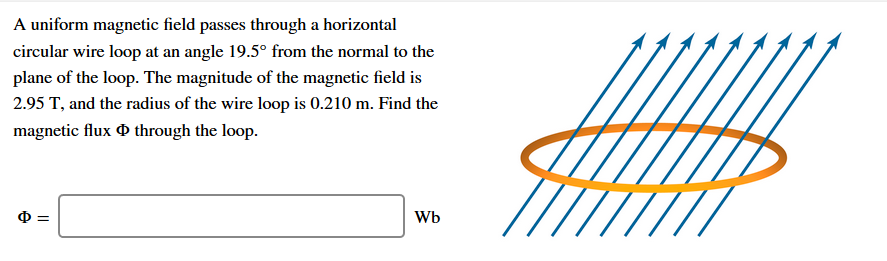A uniform magnetic field passes through a horizontal circular wire loop at an angle 19.5° from the normal to the plane of the loop. The magnitude of the magnetic field is 2.95 T, and the radius of the wire loop is 0.210 m. Find the magnetic flux through the loop. = Wb